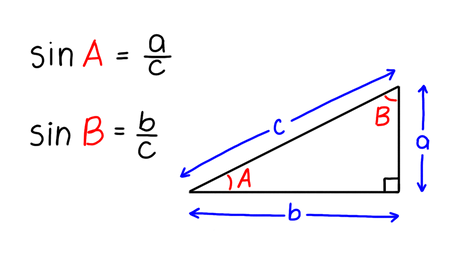VIEW IN FULL SCREEN

Edexcel GCSE Maths Sine1. Sine
2. Values and applications
3. Right-angled traingles

Sine of an angle is a ratio between the side opposite to the angle and the hypotenuse of a triangle.Sine is one of three trigonometric functions of an angle.sin(x) is calculated by calculating the ratio of two sides of a right-angled triangle, the opposite and the hypotenuse.1

Find the value of sin(A) by using the triangle shown.2

Find sinB if b = 5 and c = 25.3

Use your calculator to find the value of sin(60º).4

Which side of the triangle shown is the opposite?5

Find sinA if a = 7 cm and c = 10 cm.End of page